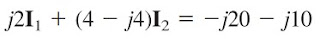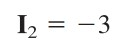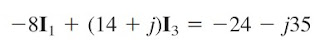omG8HI9LSKFW6lhCj8prTs0Z6lUhdOT9Jhi1Sf4m

# What is the Mesh Analysis AC Circuit Formula?

Kirchhoff's voltage law (KVL) forms the basis of mesh analysis. The validity of KVL for ac circuit can be seen in Mesh Analysis for dc circuit here. Keep in mind that the very nature of using mesh analysis is that it is to be applied to planar circuit.

## Mesh Analysis of AC Circuit

Like nodal analysis of ac circuit, we will not cover the basic explanation of its principle. You have to read the link above in order to understand 'how to use it' properly. Let us review the examples below to be able to analyze ac circuit using mesh analysis.

Determine current Io in the circuit of Figure.(1) using mesh analysis.Figure 1. Example of mesh analysis for ac circuit
Applying KVL to mesh 1, we obtain(1.1)
For mesh 2,(1.2)
For mesh 3, I3 = 5. Substituting this to Equations.(1.1) and (1.2), we obtain(1.3)(1.4)
Equations.(1.3) and (1.4) can be rewritten in matrix form as
and its determinants are
The desired current is

## Supermesh Analysis of AC Circuit

Next we will analyze an ac circuit with supermesh within it. Before doing that, make sure you have understand the principle of supermesh analysis first.

Solve for Vo in the circuit of Figure.(2) with mesh analysis.Figure 2. Example of supermesh analysis for ac circuit
As shown above, meshes 3 and 4 form a supermesh due to the current source between the meshes. For mesh 1, KVL results
or(2.1)
For mesh 2,(2.2)
For the supermesh,(2.3)
Due to the current source between meshes 3 and 4, at node A,(2.4)
We reduce the above four equations to two by elimination, instead of solving them directly.
Combining Equations.(2.1) and (2.2),(2.5)
Combining Equations.(2.2) to (2.4),(2.6)Figure 3. Analysis circuit in Figure.(2)
From Equations.(2.5) and (2.6), we obtain the matrix equation
We obtain the determinants
Current I1 is obtained as
The required voltage Vo is

Untuk Bahasa Indonesia baca Analisis Mesh Rangkaian AC
Related Posts
SHARE